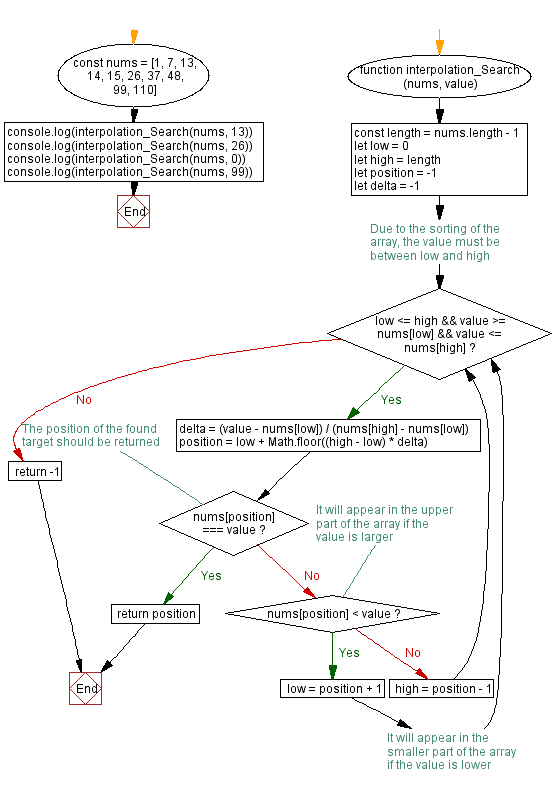# JavaScript - Interpolation algorithm

## JavaScript Searching Algorithm: Exercise-5 with Solution

Write a JavaScript program to find an element in a given sorted array of elements using Interpolation Search.

From Wikipedia:
Interpolation search is an algorithm for searching for a key in an array that has been ordered by numerical values assigned to the keys (key values). It was first described by W. W. Peterson in 1957. Interpolation search resembles the method by which people search a telephone directory for a name (the key value by which the book's entries are ordered): in each step the algorithm calculates where in the remaining search space the sought item might be, based on the key values at the bounds of the search space and the value of the sought key, usually via a linear interpolation. The key value actually found at this estimated position is then compared to the key value being sought. If it is not equal, then depending on the comparison, the remaining search space is reduced to the part before or after the estimated position. This method will only work if calculations on the size of differences between key values are sensible.

Test Data:
([1, 7, 13, 14, 15, 26, 37, 48, 99, 110], 13) -> 2
([1, 7, 13, 14, 15, 26, 37, 48, 99, 110], 26) -> 5
([1, 7, 13, 14, 15, 26, 37, 48, 99, 110], 0) -> -1
([1, 7, 13, 14, 15, 26, 37, 48, 99, 110], 99) ->8

Sample Solution:

JavaScript Code:

``````function interpolation_Search (nums, value) {
const length = nums.length - 1
let low = 0
let high = length
let position = -1
let delta = -1
// Due to the sorting of the array, the value must be between low and high
while (low <= high && value >= nums[low] && value <= nums[high]) {
delta = (value - nums[low]) / (nums[high] - nums[low])
position = low + Math.floor((high - low) * delta)
// The position of the found target should be returned
if (nums[position] === value) {
return position
}
// It will appear in the upper part of the array if the value is larger
if (nums[position] < value) {
low = position + 1
// It will appear in the smaller part of the array if the value is lower
} else {
high = position - 1
}
}
return -1
}
const nums = [1, 7, 13, 14, 15, 26, 37, 48, 99, 110]
console.log(interpolation_Search(nums, 13))
console.log(interpolation_Search(nums, 26))
console.log(interpolation_Search(nums, 0))
console.log(interpolation_Search(nums, 99))
```
```

Sample Output:

```2
5
-1
8
```

Flowchart:Live Demo:

See the Pen javascript-searching-algorithm-exercise-5 by w3resource (@w3resource) on CodePen.

* To run the code mouse over on Result panel and click on 'RERUN' button.*

Improve this sample solution and post your code through Disqus

Previous: Jump search algorithm.

What is the difficulty level of this exercise?

Test your Programming skills with w3resource's quiz.

﻿

## JavaScript: Tips of the Day

Import keyword

```// index.js
console.log('running index.js');
import { sum } from './sum.js';
console.log(sum(1, 2));

// sum.js
console.log('running sum.js');
export const sum = (a, b) => a + b;
```

With the import keyword, all imported modules are pre-parsed. This means that the imported modules get run first, the code in the file which imports the module gets executed after.
This is a difference between require() in CommonJS and import! With require(), you can load dependencies on demand while the code is being run. If we would have used require instead of import, running index.js, running sum.js, 3 would have been logged to the console.

Ref: https://bit.ly/323Y0P6

We are closing our Disqus commenting system for some maintenanace issues. You may write to us at reach[at]yahoo[dot]com or visit us at Facebook